Test: Fluid Flow Kinematics - 1

# Test: Fluid Flow Kinematics - 1 - Civil Engineering (CE)

Test Description

## 10 Questions MCQ Test GATE Civil Engineering (CE) 2023 Mock Test Series - Test: Fluid Flow Kinematics - 1

Test: Fluid Flow Kinematics - 1 for Civil Engineering (CE) 2023 is part of GATE Civil Engineering (CE) 2023 Mock Test Series preparation. The Test: Fluid Flow Kinematics - 1 questions and answers have been prepared according to the Civil Engineering (CE) exam syllabus.The Test: Fluid Flow Kinematics - 1 MCQs are made for Civil Engineering (CE) 2023 Exam. Find important definitions, questions, notes, meanings, examples, exercises, MCQs and online tests for Test: Fluid Flow Kinematics - 1 below.
Solutions of Test: Fluid Flow Kinematics - 1 questions in English are available as part of our GATE Civil Engineering (CE) 2023 Mock Test Series for Civil Engineering (CE) & Test: Fluid Flow Kinematics - 1 solutions in Hindi for GATE Civil Engineering (CE) 2023 Mock Test Series course. Download more important topics, notes, lectures and mock test series for Civil Engineering (CE) Exam by signing up for free. Attempt Test: Fluid Flow Kinematics - 1 | 10 questions in 30 minutes | Mock test for Civil Engineering (CE) preparation | Free important questions MCQ to study GATE Civil Engineering (CE) 2023 Mock Test Series for Civil Engineering (CE) Exam | Download free PDF with solutions
 1 Crore+ students have signed up on EduRev. Have you?
Test: Fluid Flow Kinematics - 1 - Question 1

### Local acceleration in fluid-flow situations exists only when

Detailed Solution for Test: Fluid Flow Kinematics - 1 - Question 1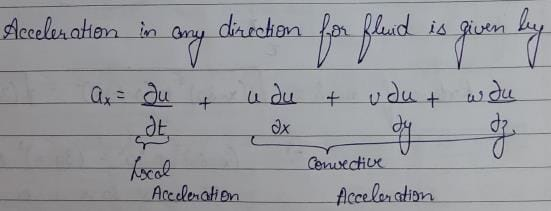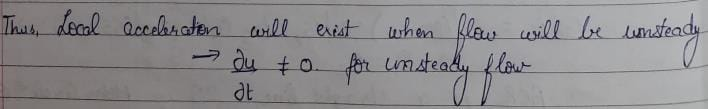Test: Fluid Flow Kinematics - 1 - Question 2

### A flownet is a graphical representation of streamlines and equipotential lines such that

Test: Fluid Flow Kinematics - 1 - Question 3

### A free vortex

Test: Fluid Flow Kinematics - 1 - Question 4

Stream lines, streak lines and path lines are all identical in case of

Test: Fluid Flow Kinematics - 1 - Question 5

In irrotational flow of an ideal fluid

Test: Fluid Flow Kinematics - 1 - Question 6

Match List-I (Format of representation) with List-ll (Context/Relevant to) and select the correct answer using the codes given below the lists: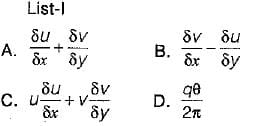List-ll
1. Relevant to a velocity potential
2. Rate of rotation about a relevant axis
3. Pressure gradient in a relevant direction
4. Continuity of flow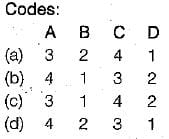Test: Fluid Flow Kinematics - 1 - Question 7

A two-dimensional flow is described by velocity components u = 2x and v = - 2y. The discharge between points (1,1) and (2, 2) is equal to

Detailed Solution for Test: Fluid Flow Kinematics - 1 - Question 7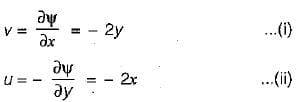Integrating (i), we get,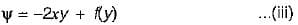Differentiating (iii) w.r.t. y, we get,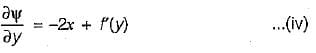Equating (ii) and (iv), we get,
f'(y) = 0    ....... (v)
Integrating (v), we get,
f(y) = C
where C is a numerical constant which can be treated as zero.
From (iii),. we get,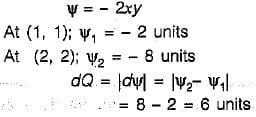Test: Fluid Flow Kinematics - 1 - Question 8

Consider the following statements in respect of two-dimensional incompressible flow with velocity components u and v in x and y directions respectively:
1. The continuity equation is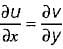2. The acceleration in x-direction is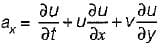3. The condition of irrotationality is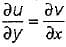4. The equation of a streamline is udy = -vdx

Which of these statements are correct?

Detailed Solution for Test: Fluid Flow Kinematics - 1 - Question 8

For two dimensional incompressible flow, :
(i) the continuity equation is'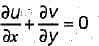(ii) the equation of a stream line is vdx - udy = 0

Test: Fluid Flow Kinematics - 1 - Question 9

An imaginary tangent at a point which shows the direction of velocity of a liquid particle at that point is

Detailed Solution for Test: Fluid Flow Kinematics - 1 - Question 9

A path-line may be defined as the line traced by a single fluid particle as it moves over a period of time, Thus a path-line will show the direction of velocity of the same fluid particle at successive instants of time. As indicated earlier a streamline on the other hand shows the direction of velocity of a number of fluid particles at the same instant of time. A fluid particle always moves tangentially to the streamline, and in the case of steady flow since there is no change in direction of the velocity vector at any point with time, the streamline is fixed in space. Therefore in steady flow the pathlines and streamlines are identical. However, in unsteady flow since the direction of velocity vector at any point may change with time, streamline may shift in space from instant to instant. A particle then follows one streamline at one instant and another at the next instant and so on, so that the path of the particle may have no resemblance to any given instantaneous streamline. In other words, in unsteady flow path-lines and streamlines are different.

Test: Fluid Flow Kinematics - 1 - Question 10

A two dimensional flow field is given by stream function ψ = x2 - y2. The magnitude of absolute velocity at a point (1, 1) is

Detailed Solution for Test: Fluid Flow Kinematics - 1 - Question 10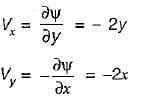at (1, 1) Vx = -2 m/s = Vy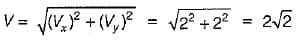## GATE Civil Engineering (CE) 2023 Mock Test Series

27 docs|296 tests
Information about Test: Fluid Flow Kinematics - 1 Page
In this test you can find the Exam questions for Test: Fluid Flow Kinematics - 1 solved & explained in the simplest way possible. Besides giving Questions and answers for Test: Fluid Flow Kinematics - 1, EduRev gives you an ample number of Online tests for practice

## GATE Civil Engineering (CE) 2023 Mock Test Series

27 docs|296 tests(Scan QR code)